# Lecture 10: Cavity-QED in the dispersive regime¶

Author: J. R. Johansson ([email protected]), http://dml.riken.jp/~rob/

The latest version of this IPython notebook lecture is available at http://github.com/jrjohansson/qutip-lectures.

The other notebooks in this lecture series are indexed at http://jrjohansson.github.com.

In :
%matplotlib inline
import matplotlib.pyplot as plt
import numpy as np

In :
from qutip import *


# Introduction¶

A qubit-resonator system can described by the Hamiltonian

$\displaystyle H = \omega_r a^\dagger a - \frac{1}{2} \omega_q \sigma_z + g (a^\dagger + a) \sigma_x$

where $\omega_r$ and $\omega_q$ are the the bare frequencies of the resonator and qubit, respectively, and where $g$ is the dipole interaction strength.

The dispersive regime occurs when the resonator and qubit is far off resonance, $\Delta \gg g$, where $\Delta = \omega_r-\omega_q$ is the detuning between the resonator and the qubit (for example $\omega_r \gg \omega_q$).

In the dispersive regime the system can be described by an effective Hamiltonian on the form

$\displaystyle H = \omega_r a^\dagger a - \frac{1}{2}\omega_q \sigma_z + \chi (a^\dagger a + 1/2) \sigma_z$

where $\chi = g^2/\Delta$ . We can view the last term as a correction of the resonator frequency that depends on the qubit state, or a correction to the qubit frequency that depends on the resonator state.

In a beautiful experiment by D. I. Schuster et al., the dispersive regime was used to resolving the photon number states of a microwave resonator by monitoring a qubit that was coupled to the resonator. This notebook shows how to simulate this kind of system numerically in QuTiP.

## Parameters¶

In :
N = 20

wr = 2.0 * 2 * pi      # resonator frequency
wq = 3.0 * 2 * pi      # qubit frequency
chi = 0.025 * 2 * pi   # parameter in the dispersive hamiltonian

delta = abs(wr - wq)        # detuning
g = sqrt(delta * chi)  # coupling strength that is consistent with chi

In :
# compare detuning and g, the first should be much larger than the second
delta/(2*pi), g/(2*pi)

Out:
(1.0, 0.15811388300841897)
In :
# cavity operators
a = tensor(destroy(N), qeye(2))
nc = a.dag() * a
xc = a + a.dag()

# atomic operators
sm = tensor(qeye(N), destroy(2))
sz = tensor(qeye(N), sigmaz())
sx = tensor(qeye(N), sigmax())
nq = sm.dag() * sm
xq = sm + sm.dag()

I = tensor(qeye(N), qeye(2))

In :
# dispersive hamiltonian
H = wr * (a.dag() * a + I/2.0) + (wq / 2.0) * sz + chi * (a.dag() * a + I/2) * sz


Try different initial state of the resonator, and see how the spectrum further down in the notebook reflects the photon distribution chosen here.

In :
#psi0 = tensor(coherent(N, sqrt(6)), (basis(2,0)+basis(2,1)).unit())

In :
#psi0 = tensor(thermal_dm(N, 3), ket2dm(basis(2,0)+basis(2,1))).unit()

In :
psi0 = tensor(coherent(N, sqrt(4)), (basis(2,0)+basis(2,1)).unit())


## Time evolution¶

In :
tlist = np.linspace(0, 250, 1000)

In :
res = mesolve(H, psi0, tlist, [], [], options=Odeoptions(nsteps=5000))


### Excitation numbers¶

We can see that the systems do not exchange any energy, because of they are off resonance with each other.

In :
nc_list = expect(nc, res.states)
nq_list = expect(nq, res.states)

In :
fig, ax = plt.subplots(1, 1, sharex=True, figsize=(12,4))

ax.plot(tlist, nc_list, 'r', linewidth=2, label="cavity")
ax.plot(tlist, nq_list, 'b--', linewidth=2, label="qubit")
ax.set_ylim(0, 7)
ax.set_ylabel("n", fontsize=16)
ax.set_xlabel("Time (ns)", fontsize=16)
ax.legend()

fig.tight_layout()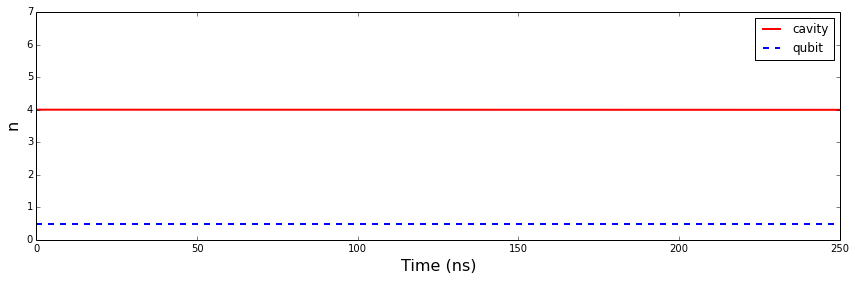However, the quadratures of the resonator are oscillating rapidly.

In :
xc_list = expect(xc, res.states)

In :
fig, ax = plt.subplots(1, 1, sharex=True, figsize=(12,4))

ax.plot(tlist, xc_list, 'r', linewidth=2, label="cavity")
ax.set_ylabel("x", fontsize=16)
ax.set_xlabel("Time (ns)", fontsize=16)
ax.legend()

fig.tight_layout()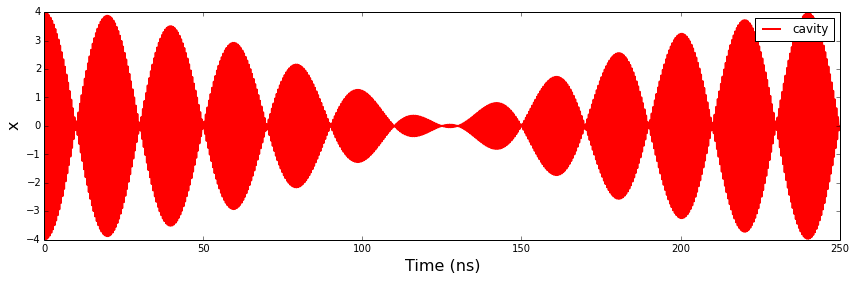### Correlation function for the resonator¶

In :
tlist = np.linspace(0, 1000, 10000)

In :
corr_vec = correlation(H, psi0, None, tlist, [], a.dag(), a)

In :
fig, ax = plt.subplots(1, 1, sharex=True, figsize=(12,4))

ax.plot(tlist, real(corr_vec), 'r', linewidth=2, label="resonator")
ax.set_ylabel("correlation", fontsize=16)
ax.set_xlabel("Time (ns)", fontsize=16)
ax.legend()
ax.set_xlim(0,50)
fig.tight_layout()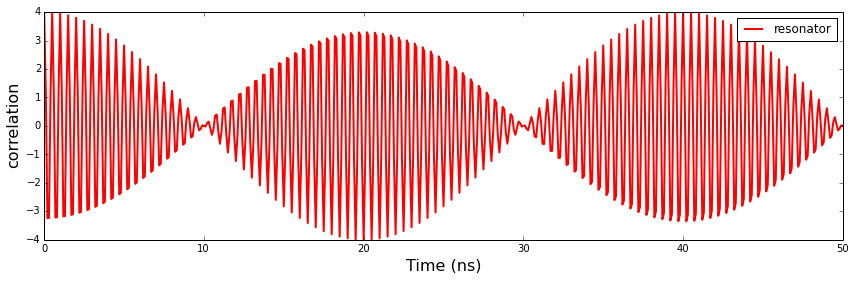### Spectrum of the resonator¶

In :
w, S = spectrum_correlation_fft(tlist, corr_vec)

In :
fig, ax = plt.subplots(figsize=(9,3))
ax.plot(w / (2 * pi), abs(S))
ax.set_xlabel(r'$\omega$', fontsize=18)
ax.set_xlim(wr/(2*pi)-.5, wr/(2*pi)+.5);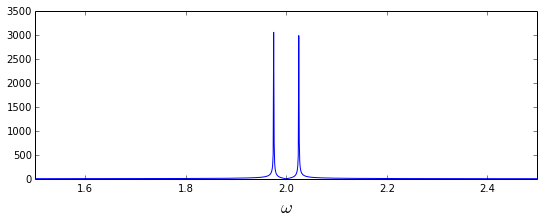Here we can see how the resonator peak is split and shiften up and down due to the superposition of 0 and 1 states of the qubit! We can also verify that the splitting is exactly $2\chi$, as expected:

In :
fig, ax = plt.subplots(figsize=(9,3))
ax.plot((w-wr)/chi, abs(S))
ax.set_xlabel(r'$(\omega-\omega_r)/\chi$', fontsize=18)
ax.set_xlim(-2,2);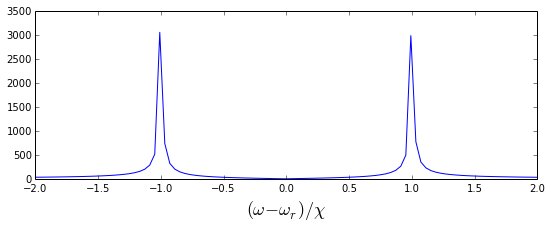### Correlation function of the qubit¶

In :
corr_vec = correlation(H, psi0, None, tlist, [], sx, sx)

In :
fig, ax = plt.subplots(1, 1, sharex=True, figsize=(12,4))

ax.plot(tlist, real(corr_vec), 'r', linewidth=2, label="qubit")
ax.set_ylabel("correlation", fontsize=16)
ax.set_xlabel("Time (ns)", fontsize=16)
ax.legend()
ax.set_xlim(0,50)
fig.tight_layout()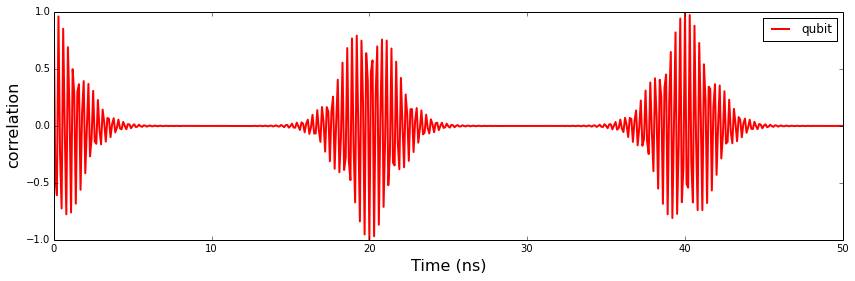### Spectrum of the qubit¶

The spectrum of the qubit has an interesting structure: from it one can see the photon distribution in the resonator mode!

In :
w, S = spectrum_correlation_fft(tlist, corr_vec)

In :
fig, ax = plt.subplots(figsize=(9,3))
ax.plot(w / (2 * pi), abs(S))
ax.set_xlabel(r'$\omega$', fontsize=18)

Out:
<matplotlib.text.Text at 0x7f172afb3da0>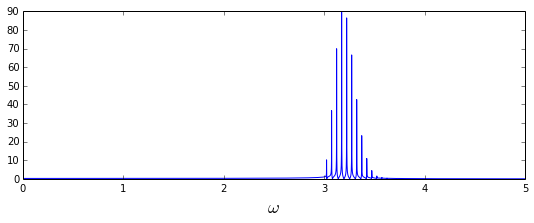It's a bit clearer if we shift the spectrum and scale it with $2\chi$

In :
fig, ax = plt.subplots(figsize=(9,3))
ax.plot((w - wq - chi) / (2 * chi), abs(S))
ax.set_xlabel(r'$(\omega - \omega_q - \chi)/2\chi$', fontsize=18)
ax.set_xlim(-.5, N);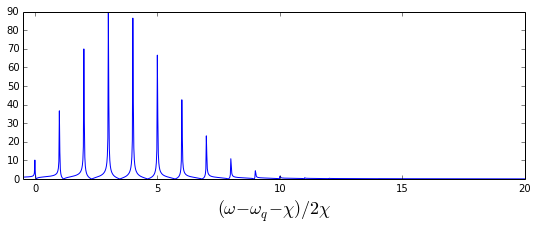Compare to the cavity fock state distribution:

In :
rho_cavity = ptrace(res.states[-1], 0)

In :
fig, axes = plt.subplots(1, 1, figsize=(9,3))

axes.bar(arange(0, N)-.4, real(rho_cavity.diag()), color="blue", alpha=0.6)
axes.set_ylim(0, 1)
axes.set_xlim(-0.5, N)
axes.set_xticks(arange(0, N))
axes.set_xlabel('Fock number', fontsize=12)
axes.set_ylabel('Occupation probability', fontsize=12);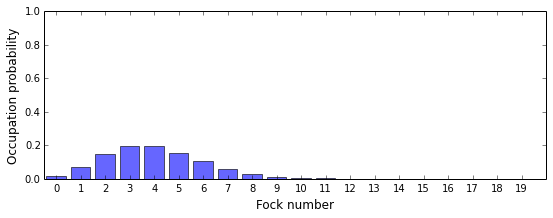And if we look at the cavity wigner function we can see that after interacting dispersively with the qubit, the cavity is no longer in a coherent state, but in a superposition of coherent states.

In :
fig, axes = plt.subplots(1, 1, figsize=(6,6))

xvec = np.linspace(-5,5,200)
W = wigner(rho_cavity, xvec, xvec)
wlim = abs(W).max()

axes.contourf(xvec, xvec, W, 100, norm=mpl.colors.Normalize(-wlim,wlim), cmap=plt.get_cmap('RdBu'))
axes.set_xlabel(r'Im $\alpha$', fontsize=18)
axes.set_ylabel(r'Re $\alpha$', fontsize=18);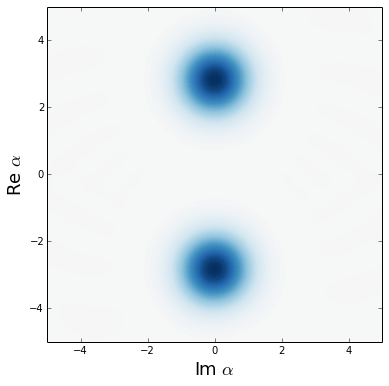### Software versions¶

In :
from qutip.ipynbtools import version_table
version_table()

Out:
SoftwareVersion
QuTiP3.0.0.dev-5a88aa8
matplotlib1.3.1
Numpy1.8.1
Cython0.20.1post0
Python3.4.1 (default, Jun 9 2014, 17:34:49) [GCC 4.8.3]
IPython2.0.0
SciPy0.13.3
OSposix [linux]
Thu Jun 26 14:20:38 2014 JST